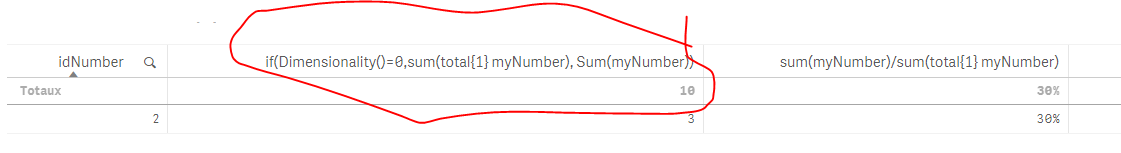# New to Qlik Sense

Discussion board where members can get started with Qlik Sense.

Announcements
Qlik® Product Spotlight: Discover what’s possible. Get more from our products.
See for yourself. Register today.
Valued Contributor

## How to calculate percentage in Qlik sense

I would like to calculate a percentage in Qlik sense. The data is as:

myNumber:

2

3

5

I would like get a table as:

myNumber   thePercentage

2                   20%

3                   30%

5                    50%

Total  10                  100%

Any suggestion is appreciated!

1 Solution

Accepted Solutions
Esteemed Contributor

## Re: How to calculate percentage in Qlik sense

sum(myNumber)/sum(total{1} myNumber) :If you want the "totaux' to always be 10 no matter your selection :change your sum(myNumber) to :

if(Dimensionality()=0,sum(total{1} myNumber), Sum(myNumber))

dimensionality()=0 is the 'total' dimension:

if we had a pivot table, and 3 dimensions: the first dimension is dimensionality()=1 and so on

Hope that helps

5 Replies
Valued Contributor

## Re: How to calculate percentage in Qlik sense

Fixed it.

Just notice Qlik sense function is case sensitive sometimes.

Esteemed Contributor

## Re: How to calculate percentage in Qlik sense

sum(myNumber)/sum(total{1} myNumber) :If you want the "totaux' to always be 10 no matter your selection :change your sum(myNumber) to :

if(Dimensionality()=0,sum(total{1} myNumber), Sum(myNumber))

dimensionality()=0 is the 'total' dimension:

if we had a pivot table, and 3 dimensions: the first dimension is dimensionality()=1 and so on

Hope that helps

Valued Contributor

## Re: How to calculate percentage in Qlik sense

Hi Omar, thanks for your help! I used a similar expression and fix it.

Valued Contributor

## Re: How to calculate percentage in Qlik sense

Please close the discussion

Valued Contributor

## Re: How to calculate percentage in Qlik sense

OK. Thanks!

Community Browser